Ex 4.4

Chapter 4 Class 12 Determinants
Serial order wise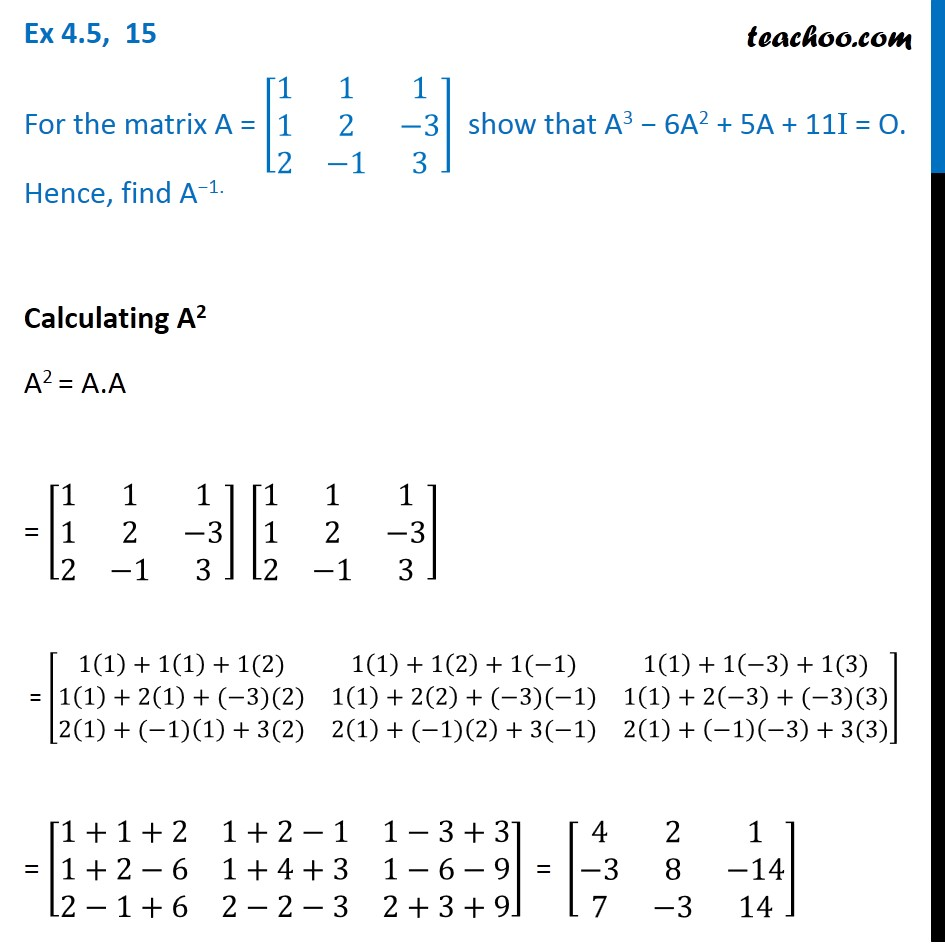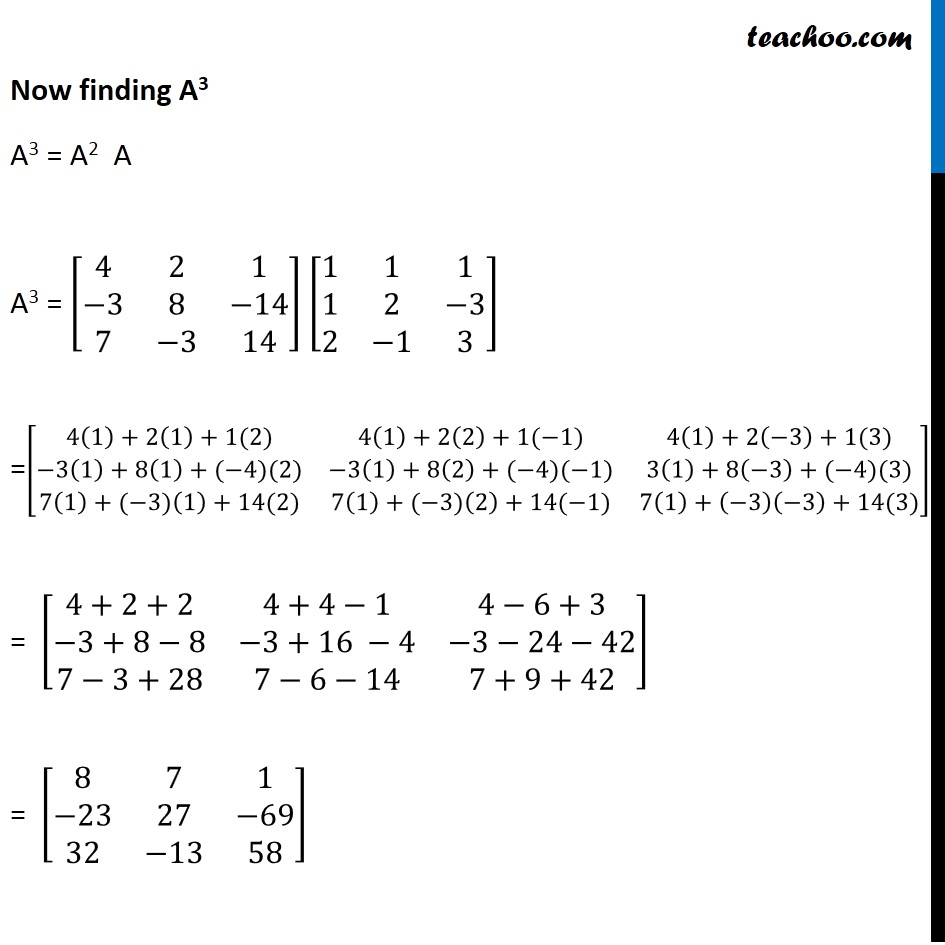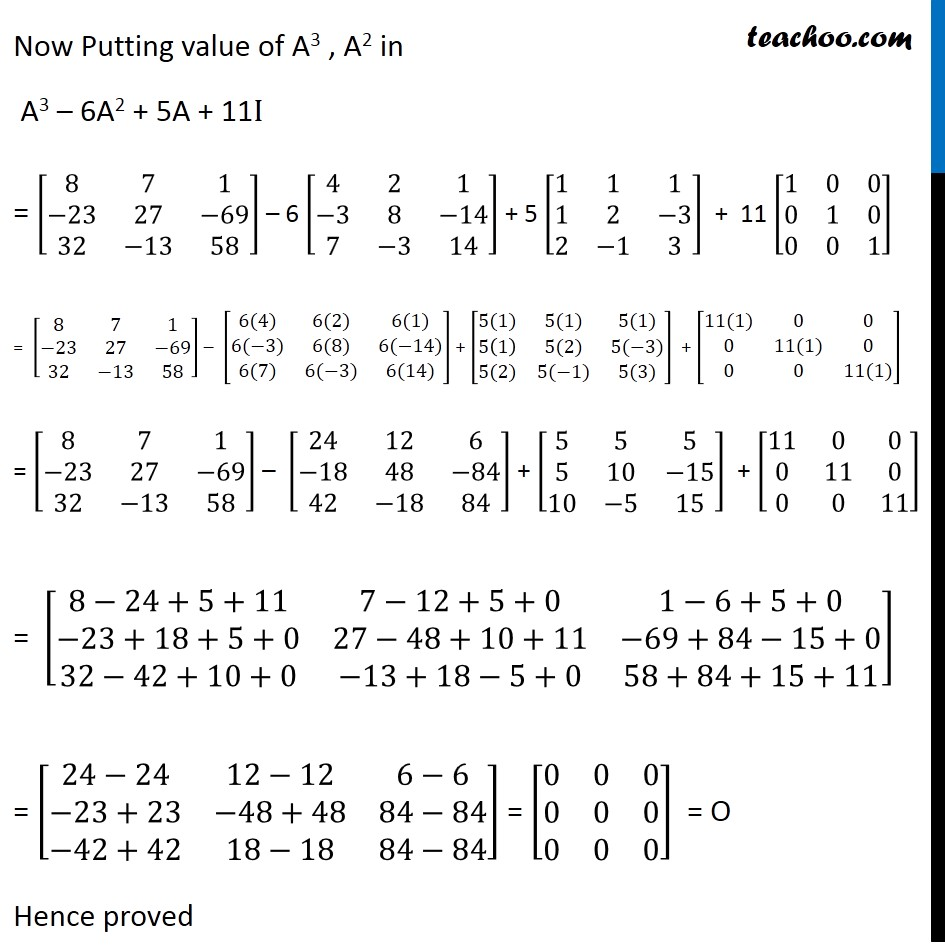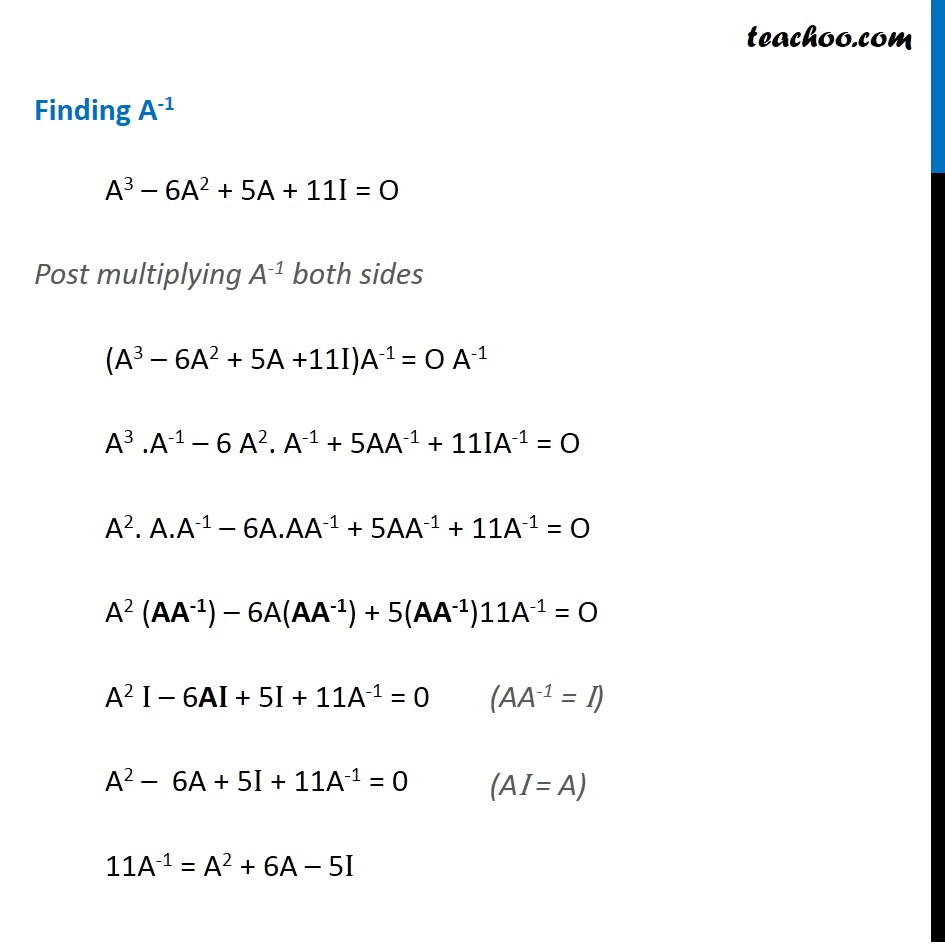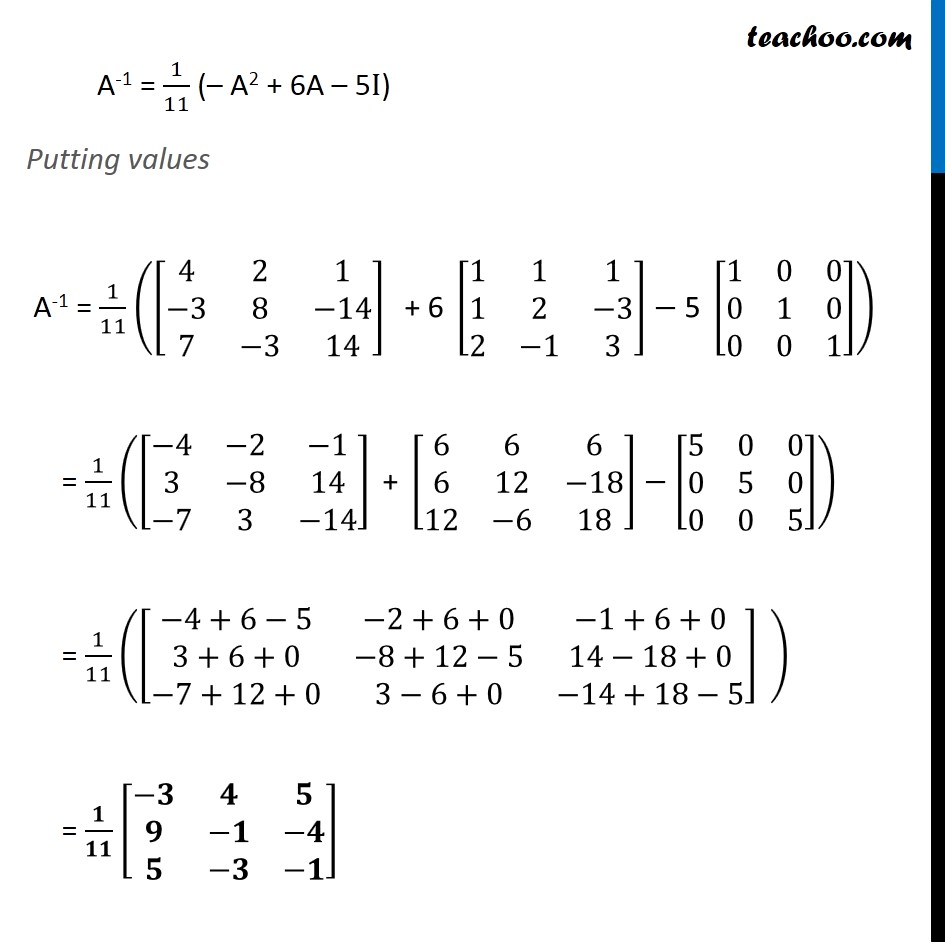Learn in your speed, with individual attention - Teachoo Maths 1-on-1 Class

### Transcript

Ex 4.4, 15 For the matrix A = [■8(1&1&[email protected]&2&−[email protected]&−1&3)] show that A3 − 6A2 + 5A + 11I = O. Hence, find A−1. Calculating A2 A2 = A.A = [■8(1&1&[email protected]&2&−[email protected]&−1&3)] [■8(1&1&[email protected]&2&−[email protected]&−1&3)] = [■8(1(1)+1(1)+1(2)&1(1)+1(2)+1(−1)&1(1)+1(−3)+1(3)@1(1)+2(1)+(−3)(2)&1(1)+2(2)+(−3)(−1)&1(1)+2(−3)+(−3)(3)@2(1)+(−1)(1)+3(2)&2(1)+(−1)(2)+3(−1)&2(1)+(−1)(−3)+3(3))] = [■8(1+1+2&1+2−1&1−[email protected]+2−6&1+4+3&1−6−[email protected]−1+6&2−2−3&2+3+9)] = [■8(4&2&1@−3&8&−[email protected]&−3&14)] Now finding A3 A3 = A2 A A3 = [■8(4&2&1@−3&8&−[email protected]&−3&14)] [■8(1&1&[email protected]&2&−[email protected]&−1&3)] =[■8(4(1)+2(1)+1(2)&4(1)+2(2)+1(−1)&4(1)+2(−3)+1(3)@−3(1)+8(1)+(−4)(2)&−3(1)+8(2)+(−4)(−1)&3(1)+8(−3)+(−4)(3)@7(1)+(−3)(1)+14(2)&7(1)+(−3)(2)+14(−1)&7(1)+(−3)(−3)+14(3))] = [■8(4+2+2&4+4−1&4−6+3@−3+8−8&−3+16 −4&−3−24−[email protected]−3+28&7−6−14&7+9+42)] = [■8(8&7&1@−23&27&−[email protected]&−13&58)] Now Putting value of A3 , A2 in A3 – 6A2 + 5A + 11I = [■8(8&7&1@−23&27&−[email protected]&−13&58)] – 6 [■8(4&2&1@−3&8&−[email protected]&−3&14)] + 5 [■8(1&1&[email protected]&2&−[email protected]&−1&3)] + 11 [■8(1&0&[email protected]&1&[email protected]&0&1)] = [■8(8&7&1@−23&27&−[email protected]&−13&58)] – [■8(6(4)&6(2)&6(1)@6(−3)&6(8)&6(−14)@6(7)&6(−3)&6(14))] + [■8(5(1)&5(1)&5(1)@5(1)&5(2)&5(−3)@5(2)&5(−1)&5(3))] + [■8(11(1)&0&[email protected]&11(1)&[email protected]&0&11(1))] = [■8(8&7&1@−23&27&−[email protected]&−13&58)] – [■8(24&12&6@−18&48&−[email protected]&−18&84)] + [■8(5&5&[email protected]&10&−[email protected]&−5&15)] + [■8(11&0&[email protected]&11&[email protected]&0&11)] = [■8(8−24+5+11&7−12+5+0&1−6+5+0@−23+18+5+0&27−48+10+11&−69+84−[email protected]−42+10+0&−13+18−5+0&58+84+15+11)] = [■8(24−24&12−12&6−6@−23+23&−48+48&84−84@−42+42&18−18&84−84)] = [■8(0&0&[email protected]&0&[email protected]&0&0)] = O Hence proved Finding A-1 A3 – 6A2 + 5A + 11I = O Post multiplying A-1 both sides (A3 – 6A2 + 5A +11I)A-1 = O A-1 A3 .A-1 – 6 A2. A-1 + 5AA-1 + 11IA-1 = O A2. A.A-1 – 6A.AA-1 + 5AA-1 + 11A-1 = O A2 (AA-1) – 6A(AA-1) + 5(AA-1)11A-1 = O A2 I – 6AI + 5I + 11A-1 = 0 A2 – 6A + 5I + 11A-1 = 0 11A-1 = A2 + 6A – 5I A-1 = 1/11 (– A2 + 6A – 5I) Putting values A-1 = 1/11 ([■8(4&2&1@−3&8&−[email protected]&−3&14)]" + 6 " [■8(1&1&[email protected]&2&−[email protected]&−1&3)]−"5 " [■8(1&0&[email protected]&1&[email protected]&0&1)]) = 1/11 ([■8(−4&−2&−[email protected]&−8&14@−7&3&−14)]" + " [■8(6&6&[email protected]&12&−[email protected]&−6&18)]−[■8(5&0&[email protected]&5&[email protected]&0&5)]) = 1/11 ([■8(−4+6−5&−2+6+0&−[email protected]+6+0&−8+12−5&14−18+0@−7+12+0&3−6+0&−14+18−5)]" " ) = 𝟏/𝟏𝟏 [■8(−𝟑&𝟒&𝟓@𝟗&−𝟏&−𝟒@𝟓&−𝟑&−𝟏)]